﻿ Numerical Investigation of an Offshore Oscillating Water ColumnPublications are Open
Access in this journal
Article Versions
Export Article
• Normal Style
• MLA Style
• APA Style
• Chicago Style
Original Article
Open Access Peer-reviewed

### Numerical Investigation of an Offshore Oscillating Water Column

Frimpong Opoku, Michael Atkinson , Mohammad Nasim Uddin
American Journal of Mechanical Engineering. 2020, 8(3), 88-105. DOI: 10.12691/ajme-8-3-1
Received August 06, 2020; Revised September 07, 2020; Accepted September 16, 2020

### Abstract

In this study, a Numerical Wave Tank (NWT) was used to investigate the flowfield of an offshore Oscillating Water Column (OWC). An inviscid solution coupled to the Volume of Fluid method was carried out using ANSYSTM Fluent to simulate six different offshore OWC configurations. The waterside chamber angle, θ1, shoreside chamber angle, θ2, and immersion extension depth d, were varied to evaluate their effect on pneumatic power and efficiency. It was determined that configuration parameters with θ1 = 45o, θ2 = 90o and d = 0 m produced the highest power with an efficiency of 23.1%; results showed that this case had a higher static pressure inside the duct which was the predominant reason for a higher pneumatic power. The cases with an additional extension into the water, d, was found to reduce the pneumatic power when compared to a similar case without an extension.

### 1. Introduction

Harvesting energy from the ocean was realized around the 18th century. The earliest patent of a wave energy converter was filed in France in 1799 1; the number of patents has increased substantially in recent times 2. The oil crises of the 1970s 3 coupled with the over-reliance on fossil fuel have led to a rebound in wave energy research. Government agencies and academic institutions are actively researching energy alternatives to supplement or replace our finite fossil fuel reserves. Increasing global energy demands require a compelling mix of sustainable energy to combat climate change challenges while satiating our energy consumption 4. The potential theoretical resource of global wave energy is around 26,000 TWh/yr 5 or 29,500 TWh/yr 6, which when harnessed efficiently would satisfy the current global energy needs 7. The amount of this theoretical resource that can be exploited has been estimated to be 10-20% of the total resource 8. Due to seasonal variation, power variability, and low efficiency of current wave energy conversion devices, R&D efforts from industry, academia and government research agencies are motivated to develop Wave Energy Converters (WECs) that are optimized, efficient, cost-competitive, and survivable; which has proven to be a challenging task 9.

A well-studied WEC is the Oscillating Water Column (OWC). An OWC consists of a structure that is submerged and open below the water surface. This configuration causes air to become trapped above the free water surface. As the water waves flow into the submerged structure, the accompanying waves causes the trapped air to oscillate and compress. The oscillating motion of the compressed air causes the air to flow through a low-pressure turbine (Wells turbine) that is coupled to an electrical generator which drives it to produce electricity. The Wells turbine has the advantage of being self-rectifying and so it does not require any rectifying valves. The added advantage of the Wells turbine is its unidirectional motion as the air flow through the turbine is bi-directional 10.

The OWC device can have a floating or fixed structure. The floating devices are designed for offshore applications where they make use of deep-water waves. These devices benefit from higher wave energy in deep oceans; however, design and installation considerations must deal with issues such as the movement of the floating OWC, electrical equipment encountering ocean water as well as underwater electrical cables that transport power to end-users. On the other hand, fixed OWCs are designed for onshore or nearshore applications. A major advantage of the onshore configuration is that electrical equipment and cables do not interact with sea water, making it easy for installation and maintenance. In contrast, the offshore configuration produces higher power since in deep-water, wave energy is not lost through friction with the seabed.

The OWC device has been a subject of several hydrodynamic studies. However, it has not been widely commercialized since the hydrodynamics of these devices are not fully understood and the technology has not attained maturity 11. Potential flow theory is often used to establish various numerical models, which tend to over-predict the hydrodynamic efficiency of these devices 11. Researchers have resorted to experimental work and Computational Fluid Dynamics (CFD) to capture the flow physics of OWC devices. Some commercial packages that are appropriate for CFD investigations of WECs include but are not limited to: ANSYSTM Fluent, CFX, Star-CD/CCM+ and FLOW-3D 12. Also, open-source or in-house codes that are used are: AMAZON, Code-Saturne, ComFLOW and OpeanFoam 12. The percentage of CFD codes that have been applied in Numerical Wave Tank (NWT) analysis of WECs in literature were reviewed by Chenari et al 10 are as follows: ANSYS Fluent (20%), CFX (11%), STAR-CCM+ (13%), FLOW-3D (7%), OpenFOAM (39%) and In-house (10%) 10.

The offshore OWC has not been a subject of many research articles compared to the onshore OWC in respect to improving the OWC efficiency. Zabihi et al., 2019 13 investigated the effect of different wave conditions, wave height, period, and wave spectra inside a fixed offshore OWC. The paper concluded that the incident wave spectrum affects the shape of the spectrum inside the OWC chamber so that for waves with low frequencies, the Pierson-Moskowitz (P-M) wave spectrum resulted in a higher device efficiency compared to when the JONSWAP spectrum is formed inside the chamber. However, when the frequency of the wave is high, and under the same conditions, the JONSWAP spectrum yielded a higher efficiency. It further concluded that the optimum device efficiency was at a wave height of 15 cm and decreased with extremely high waves. The decrease in efficiency is attributed to air leakage resulting in energy-sapping phenomena such as vortex formation at the front wall as the water waves enter the column chamber 13.

In 14, a 2D model of the offshore stationary OWC was investigated numerically using the RANS-VOF method. The investigation was carried out using 102 numerical experiments. From the results, several conclusions were drawn based on the impact of the incoming wavelength, PTO pneumatic damping and incoming wave height: 1.) the power output during inhalation and exhalation of the air in the column varies such that during the inhalation process, a shorter wavelength results in higher overall efficiency; 2.) comparison of the results show that there was very good agreement between CFD and experimental results for 3D simulation of device capture width ratio (i.e., the ratio of the power absorbed by the OWC to the wave power that is available to the device) for constant wave height and PTO damping; however, the 2D model overestimated the results 15. It was estimated that the normalized root mean square deviation is 1.9% to 5.0% 15. This highlights the capability of CFD to effectively model the performance of offshore OWC devices 15.

According to 16 the pneumatic efficiency of the OWC depends strongly on the frequency of the incident wave and the geometry of the device. It was also noted that the efficiency of the device was higher for irregular waves compared to regular waves. These results show the importance of device optimization 16.

### 2. Numerical Simulation and Wave Tank Experiment

An experimental study conducted to compare pressure variation in the wave tank was reported by Uddin et al., 2000 17. The numerical simulation of the wave tank was conducted using ANSYSTM Fluent. The results of the numerical wave tank agreed very well with the experimental results when using a very fine grid with the Volume of Fluid method and an inviscid flow solver. A comparison of the numerical solutions to the experiment is shown in Figure 1:

• Figure 1. Grid Independence study of a Numerical Wave Tank 
2.1. Basic Equations and Calculation Algorithm

In the numerical simulation, the simple harmonic angular velocity applied to the flap-type wavemaker is 17, 18:

where θ(t), h, T and S represent the simple harmonic angular velocity, water height, wave period and stroke of the flapper, respectively. Equation (1) is converted to a translational velocity and applied as a User Defined Function at the moving boundary of the numerical wave tank in ANSYSTM Fluent.

The Fluent model used to simulate the OWC consist of the mass conservation equation for the fraction of one or more phases 19 as follow: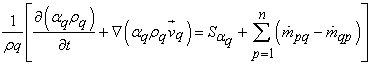(2)

where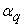= 0 when the volume is empty of qth fluid,= 1 when the volume is full of qth fluid, ρ is the density,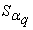is the source term which is zero in this case,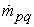is the mass transfer phase p to q and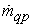is the mass transfer from phase q to p.

The unsteady, incompressible Euler equations are solved to compute the flowfield of the offshore OWC.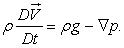(3)

The terms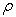,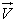, g and p represent the density of fluids, velocity field, gravitational acceleration and pressure field respectively.

The power absorbed (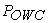) from the waves by an OWC device depends on the total pressure, velocity of air and the cross-sectional area of the duct as follows 20: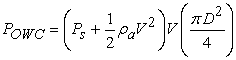(4)

where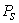,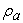, V and D represent the static pressure, density of air, air velocity and diameter of duct respectively.

The power of incident water wave (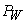) across the width of an OWC device is given by 21: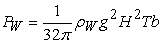(5)

where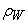, g, H, T and b represent density of water, acceleration due to gravity, wave height, wave period and width of an OWC system respectively. The efficiency of the OWC system is a measure of how much power is extracted by the device from the incoming wave. Therefore, the efficiency of an OWC system is given by: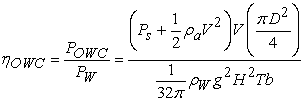(6)

where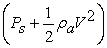term represents the average total pressure and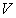the average air velocity.

2.2. Boundary Conditions

The moving wall boundary condition simulates the motion of the flap-type wavemaker 22 whose profile was set in the User Define Function (UDF) according to equation (1). The gauge pressure at the pressure outlet boundary condition is set to zero, therefore the pressure acting at the pressure outlet is atmospheric. A wall boundary condition was imposed at the surface of the duct, the sides of the column, the side opposite to the ‘moving wall’ and the bottom of the flow domain. Since the simulation was modelled as inviscid flow, the condition at the wall is a slip-condition. The symmetry boundary condition was imposed on both sides of the fluid domain to ensure mirror symmetry condition and to ensure zero-shear at the sides of the domain.

• Figure 2. Boundary Conditions of the Flow Domain

### 3. Numerical Method

The same numerical model that was used to validate the experimental results of the pressure distribution in the wave flume by 17, that is, three-dimensional unsteady Euler equations and Volume of Fluid (VOF) method with two Eulerian Phases, namely air and liquid water was used in the simulations carried out in this study. To ensure numerical stability, a fixed time step of 0.0005 seconds with a maximum of 100 iterations per time-steps was used for the simulations.

The Pressure Implicit with Splitting of Operator (PISO) was used for pressure-velocity coupling which typically works well for unsteady simulation of incompressible flow. The PISO scheme solver solves discretized momentum equation by guessing the pressure field and solving to get a velocity field, this step is called the Predictor step 23. Corrections factors are introduced to compute an updated pressure and velocity field, since the velocity field from the predictor step may not satisfy the continuity equation. These steps are repeated while updating the boundary condition until the iteration converges. For spatial discretization, the PRESTO! and Second Order Upwind scheme were used for the Pressure and Momentum equations, respectively.

3.1. Geometry

Figure 3 shows a schematic of the offshore OWC. The OWC geometry was varied by changing the angles: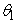,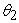and the depth, d to obtain different geometrical cases that were simulated to determine the highest power, efficiency, and location of the highest power output. The length, height and thickness of the NWT are 1.92 m, 0.22 m and 0.06 m respectively. The duct’s diameter is 0.02 m and points P1 to P5 were investigated to determine the power at each point. Each point was evenly placed at a distance of 0.04945 m. A damper with a slope of 1:5 was placed at the end of the NWT to avoid water waves from reflecting back into the flow.

• Figure 3. Schematic Diagram of an Offshore OWC
3.2. Meshing

ANSYSTM which is a commercial software that has Finite-Volume Method capabilities, was used for grid generation and the computations. A Cartesian mesh was used for the entire computational domain. A section of the meshed geometry is shown in Figure 4. The final mesh was generated using an element size of 0.005 m. The grid independence study is discussed in section 3.5.

• Figure 4. Mesh of the Offshore OWC system
3.3. Scope of Simulations

The choice of values for the angles are 30°≤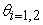≤90° in increments of 15°. Two initial cases were simulated to determine the flowfield and the power produced. The two cases are:

Case A: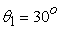,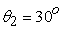with d = 0.01 m

Case B: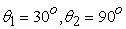with d = 0.01 m

The results of these two cases helped to determine the scope of the remaining simulation. The contour plots of the Velocity and Total Pressure for cases A and B are shown below:

Case A:,with d = 0.01 m

• Figure 5a. Contour Plot of Velocity for Case A at 60 Seconds
• Figure 5b. Contour Plot of Total Pressure for Case A at 60 Seconds

Case B:with d = 0.01m

• Figure 5c. Velocity Contour Plots of Case B at 60 Seconds
• Figure 5d. Total Pressure Contour Plot of Case B at 60 Seconds

The average power comparison for Cases A and B at 60 s considering Point 1 through to 5 is shown in Figure 6:

• Figure 6. Average Power Comparison of Case A and B

The power produced was found to be higher for Case B at all five points. These results, narrowed the scope of the simulation to geometries with θ2 = 90o while varying θ1 and d, where d represents the depth of the extension. The contour plots of the flowfield of Cases A and B is presented in Figure 5(a)-(d) for illustrations.

Finding out that Case B produced the highest power led to a focus on six cases as follows:

3.4. Simulation Time

An OWC geometry with θ1 = 30o, θ2 = 90o and d = 0.01 m was simulated for 80 s in order to estimate the required simulation time of the numerical experiment. A plot of the power versus time at points 1 to 5 is shown in Figure 7. The result indicates that the average power becomes relatively constant and the flow becomes quasi-steady for all five points at 30 seconds. Therefore, subsequent simulations to determine power output for the remaining cases was limited to 30 seconds to reduce computational expense.

• Figure 7. Plot of Average Power vs Time at Points 1 to 5
3.5. Grid Analysis

A grid independence study for Case 3 is shown in Table 2. The average power produced at point 2 was used to satisfy our grid convergence criteria of power variation of less than 5% between grids. The results showed grid convergence was achieved. This variation occurred at an element size of 0.005 m, where power output variation change between element size of 0.005 m to 0.0035 m was 4.4%. This same element size was applied to all cases in order to compare the power output.

### 4. Results and Discussions

Figure 8 presents the average power at the five points of interest in the duct. Overall, the highest power is produced for Case 3 at point 2.

• Figure 8. Plot of Average Power vs Time at Points 1 to 5
4.1. Flow-field and Plots

The biphasic nature of the flow in an experimental wave-tank is captured in the volume fraction contour plot as shown in Figure 9(a). The two phases involved in the flow are air and water. These two phases are represented with a volume fraction of 0 and 1 respectively. The plot in Figure 9(b) shows the OWC device inhaling air at 30 seconds. The inhaling and exhaling nature of the OWC system is the reason for the bi-directional flow of air. This behavior of the interface between the water and air is assumed to act as a piston. This bi-directional flow energy is utilized using a uni-directional turbine.

Any capability to increase the total pressure of the airflow from the inlet to a higher pressure at the exit of a duct will significantly contribute to an increase in pneumatic power available at the duct. The air-water interface of the OWC under investigation is at 0.08 m from the bottom of the NWT. By examining the plots of Figure 10(a), it is observed that the total pressure from height 0.1 m to 0.42 m are not significantly different for all six cases. This means that increasing the total pressure in the duct depends on the moving boundary condition which in real-world applications depend on the velocity or the dynamic pressure of the incoming waves. Comparing Figure 10(b) and 10(c) relative to Figure 10(a) shows that the contribution of the dynamic pressure to the total pressure is small. Therefore, increasing the velocity of the incoming waves will increase the total pressure and since the static pressure is not significantly higher than the total pressure further reveals that an opportunity to increase the total pressure lies in increasing the velocity of the incoming waves.

For a constant diameter duct, pneumatic power depends on the total pressure and velocity of airflow. An examination of Figure 11(a) and 11(d) shows that Case 3 has a higher total pressure along the duct compared to Case 1 while Case 1 has a higher velocity compared to Case 3. Since, Case 3 produced the highest pneumatic power, this suggests that the total pressure of the air-flow is critical to power production. Examining the static and dynamic pressure distribution in Figure 11(b) and 11(c) for their contributions to the total pressure reveals that Case 3 has a higher static pressure compared to Case 1 while the dynamic pressure for Case 1 is higher than Case 3. The average static pressure along the duct decreased by 72.62% when comparing Case 1 to 3, with Case 3 having the higher static pressure while the dynamic pressure decreased by 58.71% with Case 1 having the higher value. This shows that a higher static pressure in Case 3 was the predominant reason for having a higher pneumatic power.

• Figure 9a. Contour Plot of Volume Fraction for Case 3 at 30 Seconds
• Figure 9b. Contour Plot of Velocity for Case 3 at 30 Seconds
• Figure 10a. Total Pressure distribution from the bottom of the NWT to the Top of the Duct
• Figure 10b. Static Pressure distribution from the bottom of the NWT to the Top of the Duct
• Figure 10c. Dynamic Pressure distribution from the bottom of the NWT to the Top of the Duct
• Figure 10d. Velocity distribution from the bottom of the wave tank to the Top of the Duct
• Figure 11a. Total Pressure of air-flow from the bottom to the top of the Duct
• Figure 11b. Static Pressure of air-flow from the bottom to the top of the Duct
• Figure 11c. Dynamic Pressure of air-flow from the bottom to the top of the Duct
• Figure 11d. Velocity of air-flow from the bottom to the top of the Duct
4.2. Pneumatic Power

The pneumatic power was measured at five different points along the duct for all cases to determine which point has the highest power. It was determined for all cases that the highest power occurred at Point 2, as indicated in Figure 8. Point 2 represents halfway between the duct’s mid-point and Point 1, where Point 1 is the point at the inlet to the duct.

The average power of the six cases when computed at Point 2 showed that Case 3 with the geometry θ1 = 45o, θ2 = 90o and d = 0 m produced the highest average power of 0.005960 W as shown in Figure 14. The power versus time distributions for all six cases depicts a sinusoidal nature due to the bi-directional motion of the airflow in the column. The time series of the pneumatic power for all Cases are plotted in Figure 12(a)-(f) for illustration. Figure 13(a) shows a comparison of the power for all six cases at Point 2 with Figure 13(b) showing its moving average from 5 to 30 seconds. Since the highest moving average of the pneumatic power for Cases 1 and 3 as shown in Figure 13(b) is similar warrants the need for further investigation and is discussed in Section 4.3.

• Figure 12a. Time series of the pneumatic power for Case 1
• Figure 12b. Time series of the pneumatic power for Case 2
• Figure 12c. Time series of the pneumatic power for Case 3
• Figure 12d. Time series of the pneumatic power for Case 4
• Figure 12e. Time series of the pneumatic power for Case 5
• Figure 12f. Time series of the pneumatic power for Case 6
• Figure 13a. Time series of the pneumatic power for all Cases at Point 2
• Figure 13b. Moving average of pneumatic power from 5 to 30 seconds for all cases at Point 2
• Figure 14. Average power for all cases at Point 2
4.3. Comparison of Cases 1 and 3

The moving average smooths out the extreme fluctuations in the instantaneous parameters to show the pattern more clearly. The moving average plots of the dynamic pressure, static pressure and velocity are shown in Figure 15-17.

Figure 15 shows that Case 1 generally has a higher moving average of dynamic pressure compared to Case 3. The average dynamic pressure for Case 1 is 6.21 Pa and for Case 3 is 5.49 Pa, which supports the fact that Case 1 has a higher dynamic pressure. Figure 16 shows that the static pressure is generally higher for Case 3 compared to Case 1 which have an average static gauge pressure values of -2.06 Pa and -2.76 Pa respectively. Comparison of the moving average of the velocities as shown in Figure 17 indicates that Case 1 generally has a higher velocity compared to Case 3 with an average velocity of 2.79 m/s and 2.52 m/s respectively.

Comparing Cases 1 and 3 for parameters contributing to pneumatic power at Point 2, Case 3 has a higher value only in static pressure but generated the highest average power. This support the observation that the higher static pressure along the duct for Case 3 results in higher pneumatic power. The average pneumatic power is estimated in the OWC by considering power due to inhaling and exhaling of air as positive power since the bi-directional flow of air contributes to provide power for a uni-directional turbine.

• Figure 15. Moving average of dynamic pressure from 5 to 30 seconds at Point 2
• Figure 16. Moving average of static pressure from 2 to 30 seconds at Point 2
• Figure 17. Moving average of velocity from 5 to 30 seconds at Point 2
4.4. Analysis of Extension ‘d’ in the OWC Geometry

Cases 1-4 are used to investigate the effect of d shown in Figure 3. The d extension caused the average power produced at point 2 to decrease by 82.5% when comparing Case 1 to Case 2; by 71.5% for Case 3 to Case 4 and by 27.3% for Case 5 to Case 6.

Line 1 is drawn outside of the water column from the base of the NWT to the point of the pressure outlet as shown in Figure 18. Lines 2 to 4 are positioned from the base of the NWT to the inclined side of the OWC. These lines are inside the water column. All the lines are equally spaced at 0.08 m horizontally from each other. Comparing flow-field parameters along these lines provide information on flow-field behavior as the water flows into the column. Also, by comparing equivalent cases, it gives information on the effect of the d extension on the flow-field. Lines 1 to 5 are placed at the same location for Case 2 as shown in Figure 18.

• Figure 18. Case 1: with no d extension

4.4.1. Comparison of Cases 1 and 2

Cases 1 and 2 have the same waterside and shoreside angles. The only geometrical difference is that Case 2 has an extension d. Figure 14 shows a steep decrease in the average power produced at Point 2 for Case 2 to Case 1 comparison, giving rise to the need to investigate the reasons behind this phenomenon. Figure 19(a) shows that the water-phase generally has a higher dynamic pressure at Line 1 and decreases as the waves flow downstream to Line 5, which is closer to the point of entry of the duct. The still water height (t = 0 s) is 0.08 m measured from the bottom of the NWT. Figure 19(a) also shows that the air-phase does not have any significant change in dynamic pressure, however, it is clearly shown in Figure 19(d) that the velocity of the air-phase at Line 5 is higher compared to other locations in the column due to its proximity to the duct. Figure 19(b) and 19(c) shows that both the static and total pressure for the water phase is higher at Line 5 compared to Line 1 but at the air-phase, there is no significant difference.

Figure 20(a) reveals that Case 2 does not show a consistent pattern of decrease for the dynamic pressure from Line 1 to Line 5 as was observed in Case 1. By casually comparing Figure 19(a) and 20(a), it is shown that the d extension decreased the dynamic pressure of the flowing water on entry into the water column, however, there is no significant difference at the air-phase. Figure 20(b) and 20(c) also shows that the static and total pressure of the water-phase generally increase as the flow moves into the column but does not have any significant difference at the air-phase. Figure 20(d) reveals a significant drop in the velocity of the water-phase at the location of Line 5. A line by line comparison of the two cases is conducted below:

• Figure 19a. Plot of Dynamic Pressure (Pa) vs Y (m) for Case 1
• Figure 19b. Plot of Static Pressure (Pa) vs Y (m) for Case 1
• Figure 19c. Plot of Total Pressure (Pa) against Y (m) for Case 1
• Figure 19d. Plot of Velocity (m/s) against Y(m) for Case 1
• Figure 20a. Plot of Dynamic Pressure (Pa) vs Y (m) for Case 2
• Figure 20b. Plot of Static Pressure (Pa) vs Y (m) for Case 2
• Figure 20c. Plot of Total Pressure (Pa) vs Y (m) for Case 2
• Figure 20d. Plot of Velocity (m/s) vs Y (m) for Case 2

A line by line comparison of Cases 1 and 2 is carried out to understand the flowfield parameters. Figure 24(a) shows that at Line 1 just before the water enters into the column as shown in Figure 18, the velocity of the water at a height of 0.03 m is higher for Case 1 compared to Case 2 giving rise to a higher dynamic pressure along the same water depth as shown in Figure 21(a). The velocity of the fluid increases for Case 2 at the same line beyond the 0.03 m water height up to a depth of about 0.15 m from the bottom of the NWT, which explains why the dynamic pressure increased from the height of 0.03 m to about 0.08 m. The velocity of air becomes relatively the same from 0.15 m to 0.22 m, however, the dynamic pressure for both cases are the same at a height of about 0.10 to 0.22 m. A close observation of Figure 24(b) to 24(e) shows that inside the column, Case 1 generally has a higher fluid velocity compared to Case 2 which means that the d extension caused the dynamic pressure to be generally reduced in Case 2 as shown in Figure 21(b) to 21(d). However, Figure 21(e) shows that the dynamic pressure for Case 2 of the water-phase became higher at a depth of about 0.04 to 0.08 m.

Figure 22(a) and 23(a) shows that both the static and total pressure at Line 1 is slightly increased for the water-phase in the column of Case 2 but the air-phase has relatively the same static and total pressure. The static and total pressure distributions for line 2 to 4 for Cases 1 and 2 are similar to Figure 22(b) and 23(b) respectively. A close observation of Figure 22(b) and 23(b) shows that the d extension slightly increased both the static and total pressure inside the water column since Case 2 was observed to have a slight increase in both static and total pressure from these plots.

• Figure 21a. Dynamic Pressure Comparison of Cases 1 and 2 at Line 1
• Figure 21b. Dynamic Pressure Comparison of Cases 1 and 2 at Line 2
• Figure 21c. Dynamic Pressure Comparison of Cases 1 and 2 at Line 3
• Figure 21d. Dynamic Pressure Comparison of Cases 1 and 2 at Line 4
• Figure 21e. Dynamic Pressure Comparison of Cases 1 and 2 at Line 5
• Figure 22a. Static Pressure Comparison of Cases 1 and 2 at Line 1
• Figure 22b. Static Pressure Comparison of Cases 1 and 2 at Line 5
• Figure 23a. Total Pressure Comparison of Cases 1 and 2 at Line 1
• Figure 23b. Total Pressure Comparison of Cases 1 and 2 at Line 5
• Figure 24a. Velocity Comparison of Cases 1 and 2 at Line 1
• Figure 24b. Velocity Comparison of Cases 1 and 2 at Line 2
• Figure 24c. Velocity Comparison of Cases 1 and 2 at Line 3
• Figure 24d. Velocity Comparison of Cases 1 and 2 at Line 4
• Figure 24e. Velocity Comparison of Cases 1 and 2 at Line 5

4.4.2. Comparison of Cases 3 and 4

Cases 3 and 4 have the same geometric angles with the only difference being that Case 4 has an extension d. Figure 25(a) shows that the water-phase generally has a higher dynamic pressure at the position of Line 1 and generally decreases as the flow gets into the column. At Line 5, which is closer to the point of entry of the duct, the water-phase decreases in dynamic pressure and becomes the lowest at about water height of 0.045 to 0.06 m. Figure 25(a) also shows that the air-phase does not have any significant change in dynamic pressure, however, it is clearly shown in Figure 25(d) that the velocity of the air-phase at Line 5 is higher compared to other locations in the column due to its proximity to the duct. Figure 25(b) and 25(c) shows that both the static and total pressure for the water phase is higher at Line 5 compared to Line 1 but at the air-phase, there is no significant difference.

Figure 26(a) shows that dynamic pressure of the water-phase in Case 4 is generally decreased as the flow enters into the column but the dynamic pressure for the air-phase remains relatively the same. Figure 26(b) and 26(c) also shows that the static and total pressure of the water-phase generally increase as the flow moves into the column but does not have any significant difference at the air-phase. Figure 26(d) reveals a significant drop in the velocity of the water-phase at the location of Line 5. A line by line comparison of the two cases is conducted below:

• Figure 25a. Plot of Dynamic Pressure (Pa) vs Y (m) for Case 3
• Figure 25b. Plot of Static Pressure (Pa) vs Y (m) for Case 3
• Figure 25c. Plot of Total Pressure (Pa) vs Y (m) for Case 3
• Figure 25d. Plot of Velocity (m/s) vs Y (m) for Case 3
• Figure 26a. Plot of Dynamic Pressure (Pa) vs Y (m) for Case 4
• Figure 26b. Plot of Static Pressure (Pa) vs Y (m) for Case 4
• Figure 26c. Plot of Total Pressure (Pa) vs Y (m) for Case 4
• Figure 26d. Plot of Velocity (m/s) vs Y (m) for Case 4

Figure 28(a) and 29(a) shows that Case 3 has higher static and total pressure at Line 1 for the water-phase but the air-phase has relatively the same static and total pressure. The static and total pressure distributions for lines 2 to 4 for Cases 3 and 4 are similar to Figure 28(b) and 29(b) respectively. A close observation of Figure 28(b) and Figure 29(b) shows that the d extension slightly increased both the static and total pressure for the water-phase but for the air-phase remained relatively the same inside the column.

A line by line comparison of Cases 3 and 4 is carried out to understand the flowfield parameters of these two cases. Figure 30(a) shows that at Line 1 just before the water enters into the column, the velocity of the water at a height of 0.03 m is higher for Case 4 compared to Case 3 giving rise to a higher dynamic pressure from the base of the NWT to the same water height. The velocity of the air-phase is generally higher for Case 4 compared to Case 3 as revealed in Figure 30(a). However, the dynamic pressure of the air-phase becomes relatively the same from 0.10 to 0.22 m. Figure 30(b) shows that Case 4 has a higher velocity at Line 2 up to a water height of about 0.05 m and Case 3 was found to have a higher velocity along the rest of the line. A close observation of Figure 30(c) to 30(e) shows that inside the column, Case 3 generally has a higher fluid velocity compared to Case 4 which means that the d extension caused the dynamic pressure to be generally reduced in Case 4 as shown in Figure 27(c) to 27(e). However, Figure 27(b) shows that the dynamic pressure for Case 3 of the water-phase became higher from the bottom of the NWT to a depth of 0.05 m.

• Figure 27a. Dynamic Pressure Comparison of Cases 3 and 4 at Line 1
• Figure 27b. Dynamic Pressure Comparison of Cases 3 and 4 at Line 2
• Figure 27c. Dynamic Pressure Comparison of Cases 3 and 4 at Line 3
• Figure 27d. Dynamic Pressure Comparison of Cases 3 and 4 at Line 4
• Figure 27e. Dynamic Pressure Comparison of Cases 3 and 4 at Line 5
• Figure 28a. Static Pressure Comparison of Cases 3 and 4 at Line 1
• Figure 28b. Static Pressure Comparison of Cases 3 and 4 at Line 5
• Figure 29a. Total Pressure Comparison of Cases 3 and 4 at Line 1
• Figure 29b. Total Pressure Comparison of Cases 3 and 4 at Line 5
• Figure 30a. Velocity Comparison of Cases 3 and 4 at Line 1
• Figure 30b. Velocity Comparison of Cases 3 and 4 at Line 2
• Figure 30c. Velocity Comparison of Cases 3 and 4 at Line 3
• Figure 30d. Velocity Comparison of Cases 3 and 4 at Line 4
• Figure 30e. Velocity Comparison of Cases 3 and 4 at Line 5
4.5. Efficiency Estimation

The efficiency of the six cases are tabulated in Table 3. The average power per unit width that is available to each case according to equation (5) is 0.340923 W since all cases were exposed to the same flow parameters such as wave height and period. The power of incident wave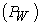across the width of an OWC device is the same for all the cases, since they all have the same width. As indicated in Table 3 below, Case 3 which produced the highest power has the highest efficiency at 29.1%.

### 5. Conclusions

Three-dimensional unsteady Euler simulations were carried out to investigate potential geometry optimization characteristics of an offshore OWC device. A NWT was developed using ANSYSTM Fluent to determine the maximum average power and efficiency of six OWCs. It was found that Case 3 with θ1 = 45o, θ2 = 90o and d = 0 m produced the maximum average power and the highest efficiency. The geometries with a d extension such as Cases 2, 4 and 6 had a decreased pneumatic power produced compared to same geometries without a d extension. The extension generally decreased the velocities of the air- and water-phases inside the water column, also the dynamic pressure of the water-phase was generally reduced as a result of the extension but was relatively the same for the air-phase. Case 4 showed an increase in the static and total pressure in the water-phase but remained relatively the same for the air-phase due to the d extension. However, inside the duct where an air turbine is placed, the total pressure is higher for Case 1 compared to Case 2 and also higher for Case 3 compared to Case 4. This indicates that the d extension results in lower total pressure inside the duct. Similarly, a reduction in velocity for Cases 2, 4 and 6 produced lower average powers compared to geometries without a d extension.

As part of our future work, we plan to conduct experimental studies of the OWC geometries to verify our numerical findings and explore higher-fidelity, 3D Detached Eddy Simulation (DES) to better understand OWC flow topology.

### Acknowledgements

This research was partially supported by the North Carolina Ocean Renewable Energy Program.

### Nomenclature

b width of an OWC system, m

d depth of extension, m

D diameter of duct, m

g gravitational acceleration,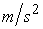h water height, m

H wave height, mmass transfer phase p to qmass transfer from phase q to p.

p pressure field, Pastatic pressure, PaPneumatic power in the duct of the OWC, Wpower of incident water wave across the width of an OWC device, W

S stroke of the flapper, msource term

T wave period, svelocity field,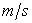average air velocity,θ(t) simple harmonic angular velocity, rad/s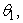geometrical inclination of geometry,

ρ density of fluid,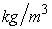density of air,density of water,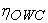efficiency of an OWC system

### References

  Ross, D., “Power from Sea Waves,” Oxford: Oxford University Press. 1995. In article  Falcao, A. F. O., “Wave energy utilization: A review of the technologies”, Renewable and Sustainable Energy Reviews, Elsevier, 14(2010). 899-900. Oct. 2009. In article View Article  Giovanni, C., “The first oil shock, stylized facts, reflections and the easterly puzzle in a forty-year retrospective, Munich Personal RePEc Archive, 4-9. Aug. 2014. In article  OECD and IEA, “OECD Green Growth Studies: Energy”, Preliminary Version. 9-20. 2011. In article  Mork, G., Barstow, S., Kabuth, A., Pontes, M.T., “Assessing the global wave energy potential,” In: Proceedings of the OMAE2010 (ASME), 29th International Conference on Ocean, Offshore Mechanics and Arctic Engineering, Shanghai, China. 447-454. June 2010. In article View Article  Aderinto, T. and Li, H., “Ocean Wave Energy Converters: Status and Challenges”, Energies MDPI. 1. May 2018. In article View Article  Guedes Soares, C., Bhattacharjee, J. and Karmakar, D., “Overview and Prospects for Development of Wave and Offshore Wind Energy,” 65(2), 88. Feb. 2014. In article  Cruz, J., “Ocean Wave Energy: Current Status and Future Perspective”, Springer Science and Business Media: Berlin/Heidelberg, Germany. 2007. In article  Windt, C., Davidson, J. and Ringwood, J. V., “High-fidelity numerical modelling of ocean wave energy systems: A review of computational fluid dynamics - based numerical wave tanks,” Renewable and Sustainable Energy Reviews, Elsevier, 610. May 2018. In article View Article  Chenari, B., Saadatian, S.S., Ferreira, A., “Wave Energy Systems: An overview of different wave energy converters and recommendation for future improvement” Eight International Technology, Education and Development Conference, Valencia, Spain. March 2014. In article  Singh, U., Abdussaime, N., and Hore, J., “Hydrodynamic performance of a floating offshore OWC wave energy converter: An experimental study,” Renewable and Sustainable Energy Reviews, Elsevier. 117 (2020). Oct. 2019. In article View Article  Mingham, C., Qian, L., and Causon, D., “Computational Fluid Dynamics (CFD) Models”, In: Numerical Modelling of Wave Energy Converters - State-of-the-art techniques for single devices and arrays. Elsevier Inc. 105-113. 2016. In article View Article  Zabihi, M., Mazaheri, S. and Namin, M. M., “Experimental hydrodynamic investigation of a fixed offshore Oscillating Water Column device,” Applied Ocean Research, Elsevier, 85(2019). 20-33. Feb 2019. In article View Article  Elhanafi, A., Fleming, A., Macfarlane, G., and Leong, Z., “Numerical hydrodynamic analysis of an offshore stationary - floating oscillating water column - wave energy converter using CFD,” International Journal of Naval Architecture and Ocean Engineering, Elsevier, 9(2017). 77-79. Oct. 2016. In article View Article  Elhanafi, A., and Kim, C. J., “Experimental and numerical investigation on wave height and power take-off damping effects on the hydrodynamic performance of an offshore-stationary OWC wave energy converter,” Renewable Energy, Elsevier, 125(2018). 518-528. March 2018. In article View Article  Crema, I., Simeonetti, I., Cappietti, L., and Oumeraci, H., “Laboratory experiments on Oscillating Water Column wave energy converters integrated in a very large floating structures,” Proceedings of the 11th European Wave and Tidal Energy Conference (EWTEC). Nantes, France. Sept. 2015. In article  Uddin, M. N., Atkinson, M., Opoku, F. “A Computational Fluid Dynamics Investigation of a Numerically Simulated Wave Tank,” American Journal of Mechanical Engineering, vol. 8, no. 1 (2020): 40-49. 40-49. May 2020. In article  Anbarsooz, M., Passandideh-Farad, M., and Moghiman, M., “Fully Nonlinear Viscous Wave Generation in Numerical Wave Tanks,” Elsevier, Ocean Engineering, 59(2013), 73-85. Nov. 2012. In article View Article  Gomes, M. N., Olinto, C. R., Rocha, L. A. O., Souza, J.A. and Isoldi, L. A. “Computational Modelling of a Regular Wave Tank,” Engenharia Termica (Thermal Engineering), 8(01), 44-50. June 2009. In article View Article  Dizadji, N., and Sajadian, S. E., “Modeling and Optimization of the chamber of OWC system”, Energy, Elsevier, 36(2011). 2362. Apr 2011. In article View Article  Mahnamfar, F. and Altunkaynak, A., “Comparison of numerical and experimental analyses for optimizing the geometry of OWC systems”. Ocean Engineering, Elsevier, 130(2017), 10-24. Nov. 2016. In article View Article  Feng, X. and Wu, W., “Generation of Water Waves Using Momentum Source Wave-Maker Applied to a RANS Solver”. Hindawi - Mathematical Problems in Engineering. 1-11. May 2019. In article View Article  Ye, S., Lin, Y., Xu, L. and Wu, J., “Improving Initial Guess for the Iterative Solution of Linear Equation Systems in Incompressible Flow”. Mathematics MDPI. 1-20. Jan. 2020. In article View ArticleThis work is licensed under a Creative Commons Attribution 4.0 International License. To view a copy of this license, visit http://creativecommons.org/licenses/by/4.0/